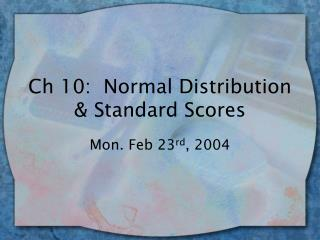DownloadDownload PresentationCh 10: Normal Distribution & Standard Scores

# Ch 10: Normal Distribution & Standard Scores

Télécharger la présentation## Ch 10: Normal Distribution & Standard Scores

- - - - - - - - - - - - - - - - - - - - - - - - - - - E N D - - - - - - - - - - - - - - - - - - - - - - - - - - -
##### Presentation Transcript

1. Ch 10: Normal Distribution & Standard Scores Mon. Feb 23rd, 2004

2. Normal Distribution (ND) • Common theoretical distribution w/bell-shaped, symmetrical curve • Mean, median, mode all occur at peak • Properties of the ND: • Area under it = 1.0 (100% scores) • 50% scores fall above midpt, 50% below • Betw Mean & + or - 1 SD = 34.13% of scores • Betw 1 & 2 SD (pos or neg) = 13.59% of scores • Beyond 2 SD (pos or neg) = 2.28% of scores • …or 68-95-99.7 rule (68% between mean and +/- 1 SD; 95% betw mean and +/- 2 SD; 99.7% betw mean and +/- 3 SD.

3. Z scores • By remembering these %s, if we have a ND, can determine % scores falling betw any score & the mean • Use of z scores (standardized scores) • The difference betw any score & the mean in standard deviation units Z = (y – ybar) / Sy • Notice sign & magnitude of z score…

4. (cont.) • If pos z score, raw score was above the mean; if neg z score  below the mean • Magnitude indicates how many standard deviations the score is away from the mean • SAT mean = 500, Sy = 100, your score (y) = 620 Z = 620 – 500 / 100 = 1.2 You scored 1.2 standard deviations above the mean Could compare to your friend, y = 540 Z = 540 – 500 / 100 = .4 (scored 4/10’s of standard deviation above mean)

5. Converting z to y • May also need to transform z to raw score: Y = Ybar + Z(Sy) Someone tells you they scored 2 SD above the mean SAT; what raw score? Y = 500 + 2(100) = 700 SAT score

6. Standard Normal Table (aka Unit Normal Table) • Table showing proportion of scores corresponding to a certain z score • Appendix B in book: 3 columns • Col A gives z scores (all pos since symmetrical – just find same z for neg) • Col B gives proportion of scores betw the z score & mean • Col C gives proportion of scores beyond the z score

7. Using the table… • Start by sketching distribution, label mean & Sy, then score you’re interested in • Shade relevant area of distribution • Translate y into a z score, use table • Examples: IQ test has ybar=100, Sy = 15 • What is probability of having IQ < 85? >130? • What IQ score is needed to be in top 5%? • What is probability of having IQ between 90 &120?

8. Lab 11 • Click button for “ND” demo, move flags & find % associated w/scores • Use unit normal table (either link from lab or your book)…note how the tables differ slightly. • SPSS – lab11.sav dataset…create z scores (follow directions in lab)